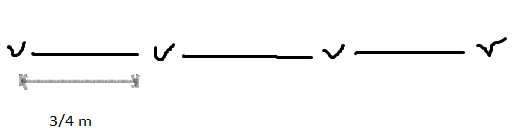Courses
Courses for Kids
Free study material
Free LIVE classes
MoreLIVE
Join Vedantu’s FREE Mastercalss

# Saili plants 4 saplings in a row, in her garden. The distance between two adjacent saplings is $\dfrac{3}{4}$ m. Find the distance between the first and the last sapling.Verified
361.8k+ views
Hint: We just have to find the distance between the first and the last sapling. So what we have to do is that, we have to find the total number of gaps between 4 saplings and multiply it with the distance between the adjacent saplings.

Before proceeding with the question, we must know how to plot the figure showing the saplings that are given in the question. Here, we have been given that Saili plants 4 saplings in a row in her garden. The distance between two adjacent saplings is also given to us as $\dfrac{3}{4}$m.
Using this data, we can plot the arrangement of the saplings in Saili’s garden as shown as below:Therefore, we can see that there are a total of 3 spaces between the four saplings and the distance between the adjacent saplings is $\dfrac{3}{4}$ m.
As we know the distance between adjacent saplings, we can calculate the distance between the first and the last sapling. This can be done by multiplying the number of spaces, i.e. 3 and the distance between adjacent saplings, i.e. $\dfrac{3}{4}$.
Therefore, we get,
$\dfrac{3}{4}\left( 3 \right)=\dfrac{9}{4}$
Hence, the distance between the first and the last sapling is $\dfrac{9}{4}$ m.

Note: This question is a direct question which requires the understanding of the way the saplings are placed. It is necessary to draw the figure and consider the number of spaces. The mistake which could be committed is by not drawing the figure and taking the number of spaces as 4 instead of 3. We have to be careful that if there are 4 saplings then there will be only 3 gaps between the 4 saplings.
Last updated date: 30th Sep 2023
Total views: 361.8k
Views today: 6.61k